Arduino

# RGB Camera Backlight Control With Android MobileFebruary 20, 2018 by DIY Partners
Share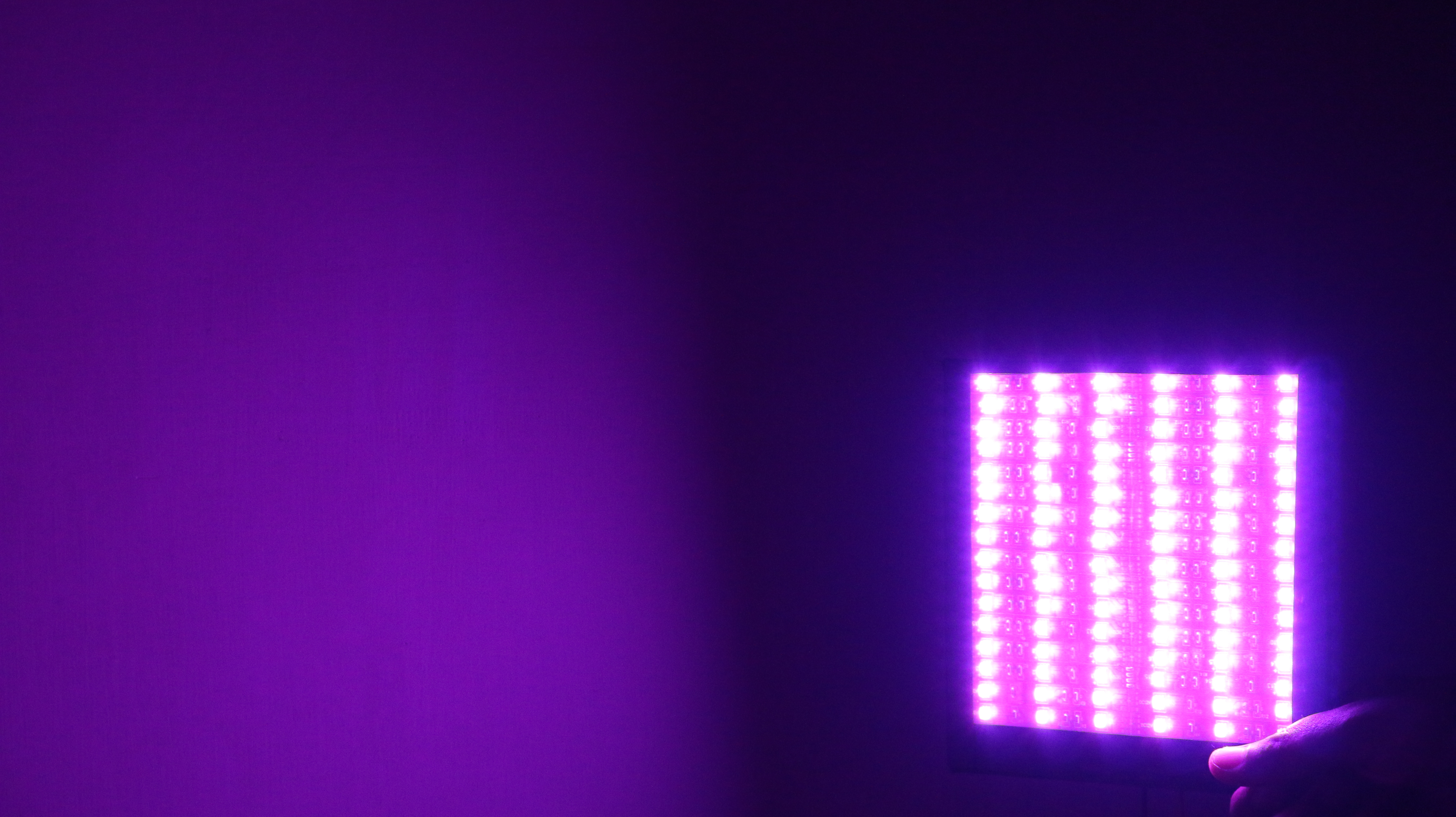RGB Lights Compaing Three LED Lights Red,Green & Blue. We Adjusting the Brightness Of the LED it Create new Colour. So t

## Others

 1 Arduino uno 1 arduino nano 1 bluetooth hc 06 1 RGB LED

RGB Lights Compaing Three LED Lights Red,Green & Blue. We Adjusting the Brightness Of the LED it Create new Colour. So the LED Adjusting Brightness using Codes(0-255).

Mixing colors

â–ºTo produce other colors, you can combine the three colors in different intensities. To generate different colors you can use PWM to adjust the brightness of each LED.

â–ºAs the LEDs are very close to each other, we can only see the final colors result rather than the three colors individually.

â–ºTo have an idea on how to combine the colors, take a look at the following chart. This is the simplest color mixing chart, there are more complex color charts on the web.

â–ºRGB LEDs have 4 pins which can be distinguished by their length. The longest one is the ground (-) or voltage (+) depending if it is a common cathode or common anode LED, respectively.RGB LED

RGB LED is acombination of 3 LEDs in just one package

Â· 1x Red LED

Â· 1x Green LED

Â· 1x Blue LED

The color produced bythe RGB LED is a combination of the colors of each one of these three LEDs.

## Mixing colors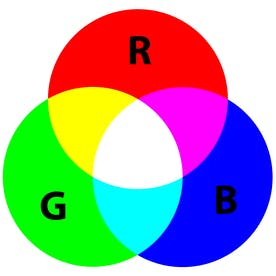MIXING Color

To produce other colors, you can combine the three colors in different intensities. To generate different colors you can use PWM to adjust the brightness of each LED. As the LEDs are very close to each other, we can only see the final colors result rather than the three colors individually.

R G B

(255,255,255)= White color

The 255 is full brightness of the led light

## RGB LED Two Types: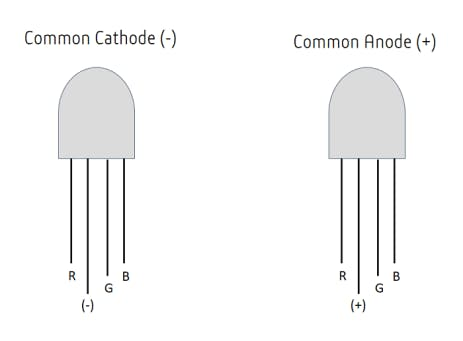Type of led``````int redPin = 11;
int greenPin = 10;
int bluePin = 9;

void setup()
{
pinMode(redPin, OUTPUT);
pinMode(greenPin, OUTPUT);
pinMode(bluePin, OUTPUT);
}

void loop()
{
setColor(255, 0, 0);  // red
delay(1000);
setColor(0, 255, 0);  // green
delay(1000);
setColor(0, 0, 255);  // blue
delay(1000);
setColor(255, 255, 0);  // yellow
delay(1000);
setColor(80, 0, 80);  // purple
delay(1000);
setColor(0, 255, 255);  // aqua
delay(1000);
}

void setColor(int red, int green, int blue)
{
#ifdef COMMON_ANODE
red = 255 - red;
green = 255 - green;
blue = 255 - blue;
#endif
analogWrite(redPin, red);
analogWrite(greenPin, green);
analogWrite(bluePin, blue);
}``````

## ARDUINO UNO USING BLUETOOTH RGB CONTROLLER: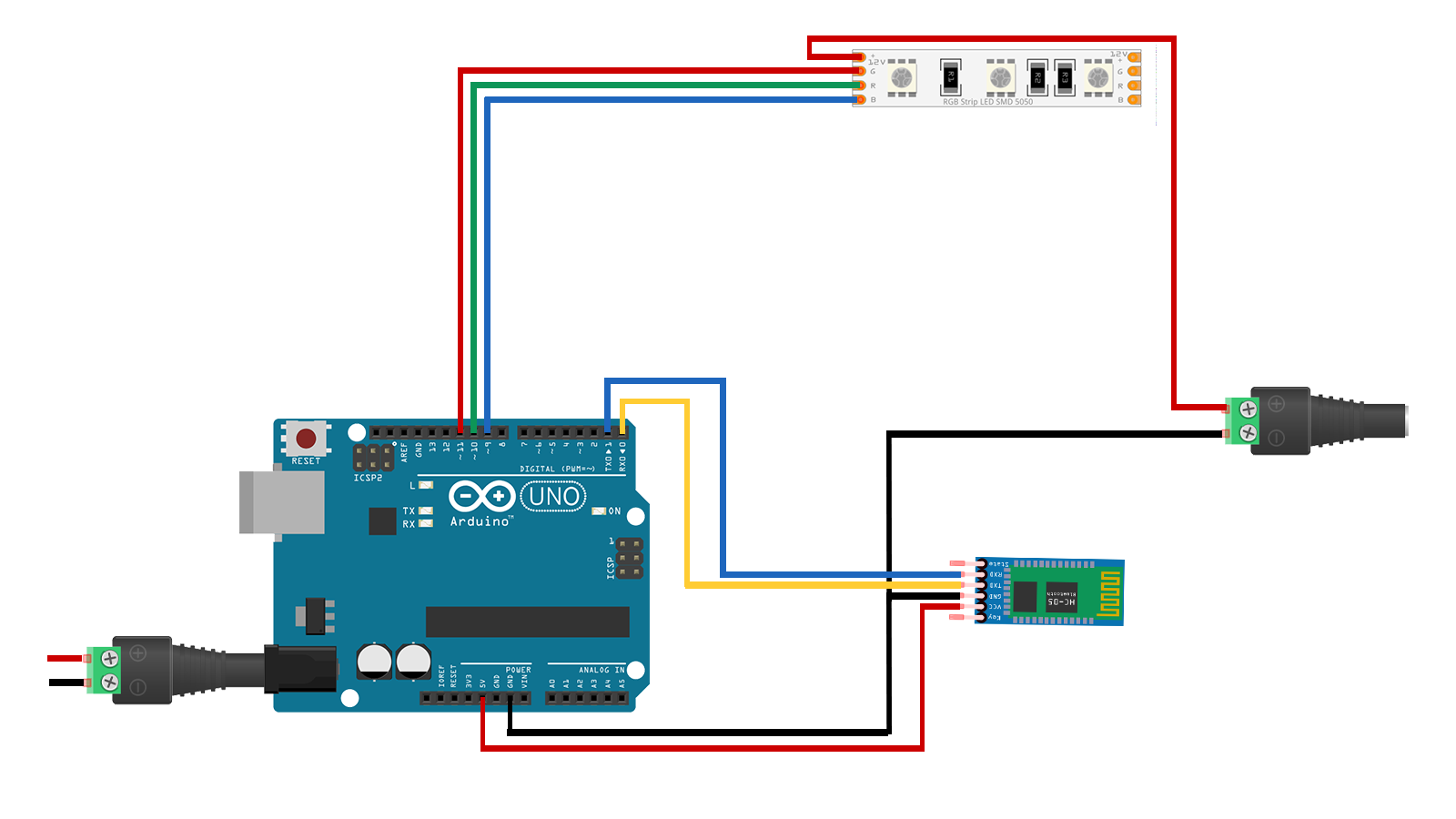``````int color =0;
int red = 12;
int green =11;
int blue =10;

void setup() {
Serial.begin(9600);
pinMode(red, OUTPUT);
pinMode(green, OUTPUT);
pinMode(blue, OUTPUT);

analogWrite(red,0);
analogWrite(green,0);
analogWrite(blue,0);
}

void loop(){
if(Serial.available()>0){
char Rec = char(color);
if (Rec != '0')
{
Serial.println(Rec);
}
}

//Black
if (color == 'B')
{
analogWrite(red,0);
analogWrite(green,0);
analogWrite(blue,0);
}

//WHITE
if (color == 'W')
{
analogWrite(red,255);
analogWrite(green,255);
analogWrite(blue,255);
}

//RED
if (color == 'R')
{
analogWrite(red,255);
analogWrite(green,0);
analogWrite(blue,0);
}

//LIME
if (color == 'L')
{
analogWrite(red,0);
analogWrite(green,255);
analogWrite(blue,0);
}

//BlUE
if (color == 'E')
{
analogWrite(red,0);
analogWrite(green,0);
analogWrite(blue,255);
}

//Yellow
if (color == 'Y')
{
analogWrite(red,255);
analogWrite(green,255);
analogWrite(blue,0);
}

//Cyan/Aqua
if (color == 'C')
{
analogWrite(red,0);
analogWrite(green,255);
analogWrite(blue,255);
}

//Magenta / Fuchsia
if (color == 'M')
{
analogWrite(red,255);
analogWrite(green,0);
analogWrite(blue,255);
}

// Maroon
if (color == 'F')
{
analogWrite(red,128);
analogWrite(green,0);
analogWrite(blue,0);
}

// Olive
if (color == 'O')
{
analogWrite(red,128);
analogWrite(green,128);
analogWrite(blue,0);
}

//Green
if (color == 'G')
{
analogWrite(red,0);
analogWrite(green,128);
analogWrite(blue,0);
}

// Purple
if (color == 'P')
{
analogWrite(red,128);
analogWrite(green,0);
analogWrite(blue,128);
}

//  Navy
if (color == 'N')
{
analogWrite(red,0);
analogWrite(green,0);
analogWrite(blue,128);
}

//light coral
if (color == 'J')
{
analogWrite(red,240);
analogWrite(green,128);
analogWrite(blue,128);
}

//orange red
if (color == 'X')
{
analogWrite(red,255);
analogWrite(green,69);
analogWrite(blue,0);
}

//green yellow
if (color == 'G')
{
analogWrite(red,173);
analogWrite(green,255);
analogWrite(blue,47);
}

// spring green
if (color == 'S')
{
analogWrite(red,0);
analogWrite(green,255);
analogWrite(blue,127);
}

//   aqua marine
if (color == 'A')
{
analogWrite(red,127);
analogWrite(green,255);
analogWrite(blue,212);
}

//   hot pink
if (color == 'H')
{
analogWrite(red,255);
analogWrite(green,105);
analogWrite(blue,180);
}

// honeydew
if (color == 'D')
{
analogWrite(red,240);
analogWrite(green,255);
analogWrite(blue,240);
}

// light gray / light grey
if (color == 'U')
{
analogWrite(red,211);
analogWrite(green,211);
analogWrite(blue,211);
}
}``````

## ARDUINO NANO USING BLUETOOTH RGB CONTROLLER: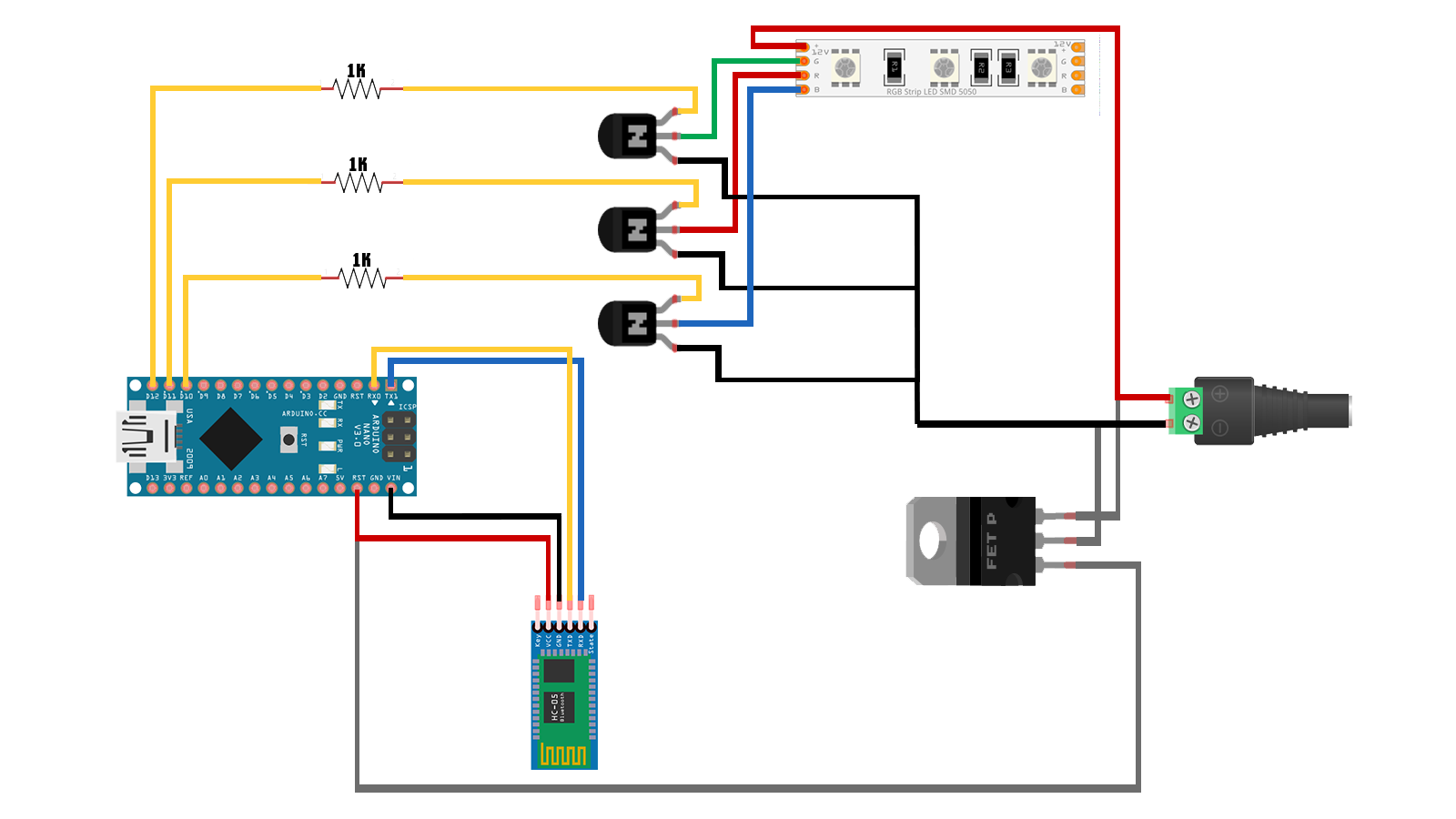http://gestyy.com/wvr2kw

``// Your code here``